Examples

Chapter 8 Class 12 Application of Integrals
Serial order wise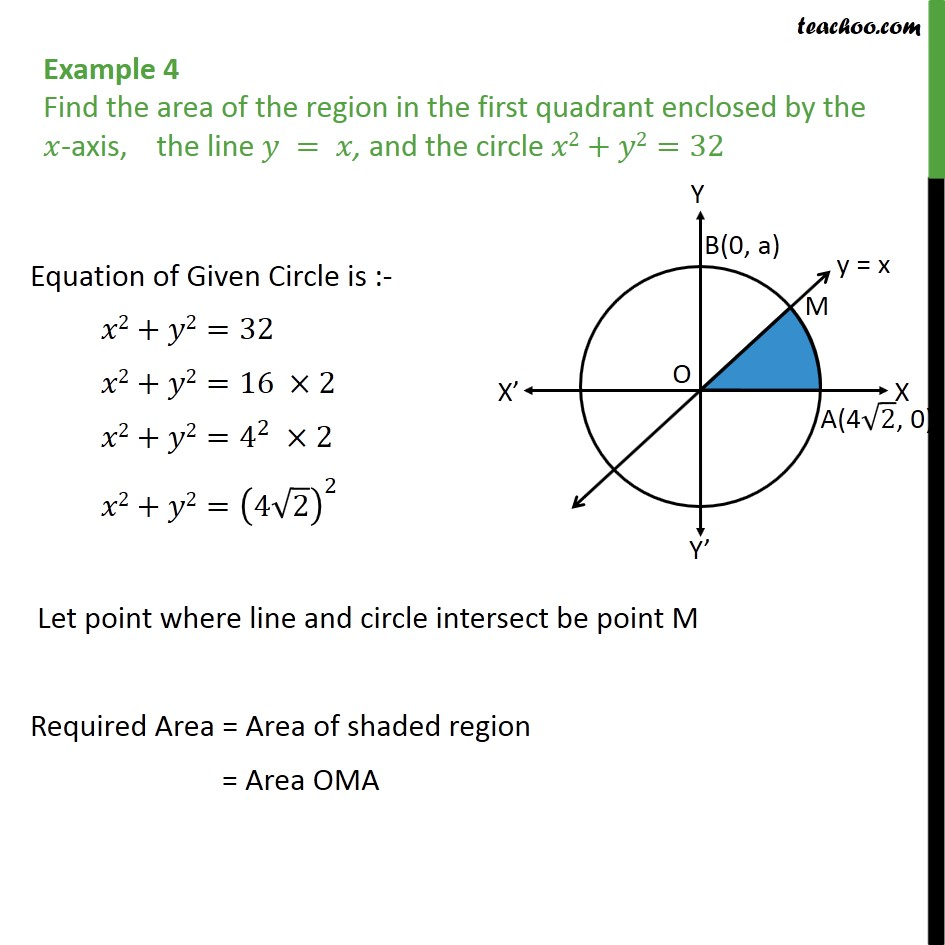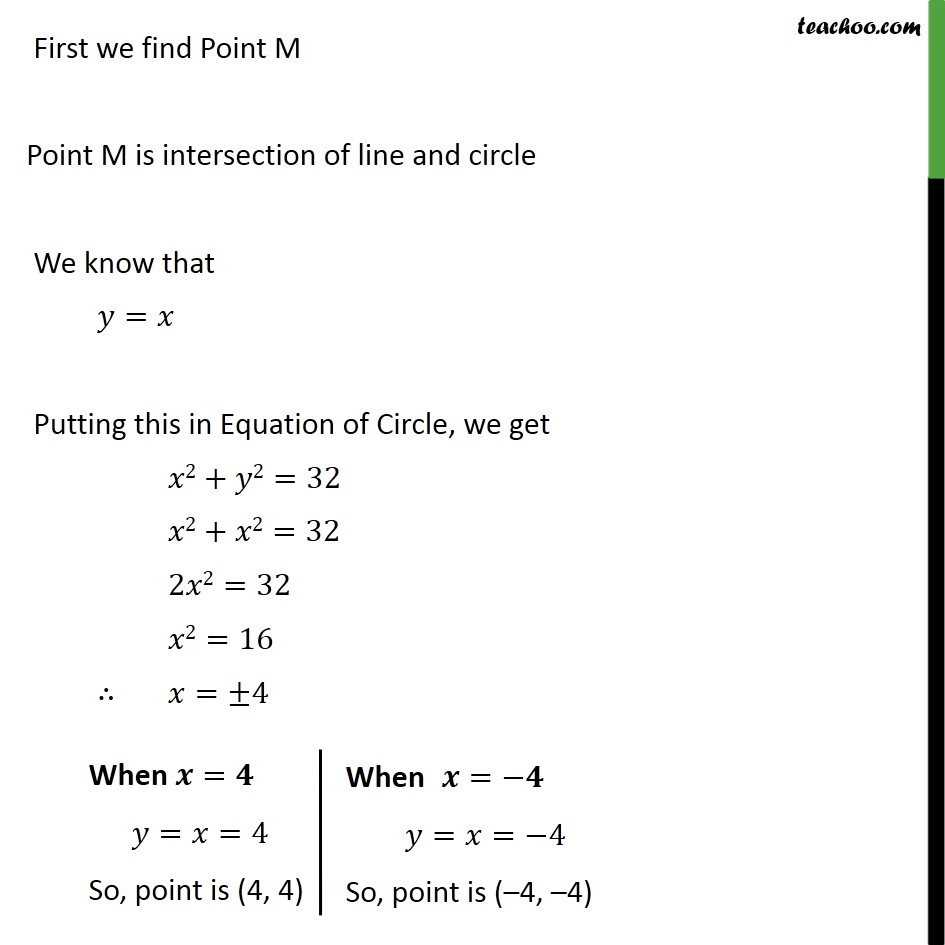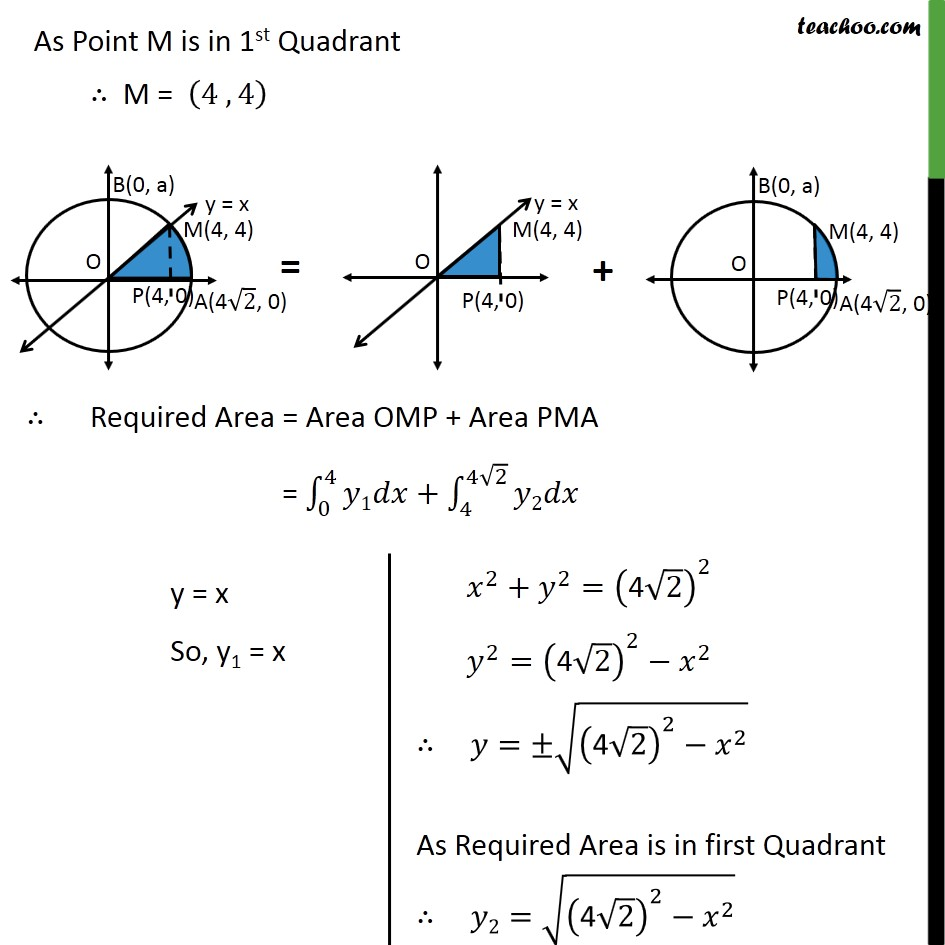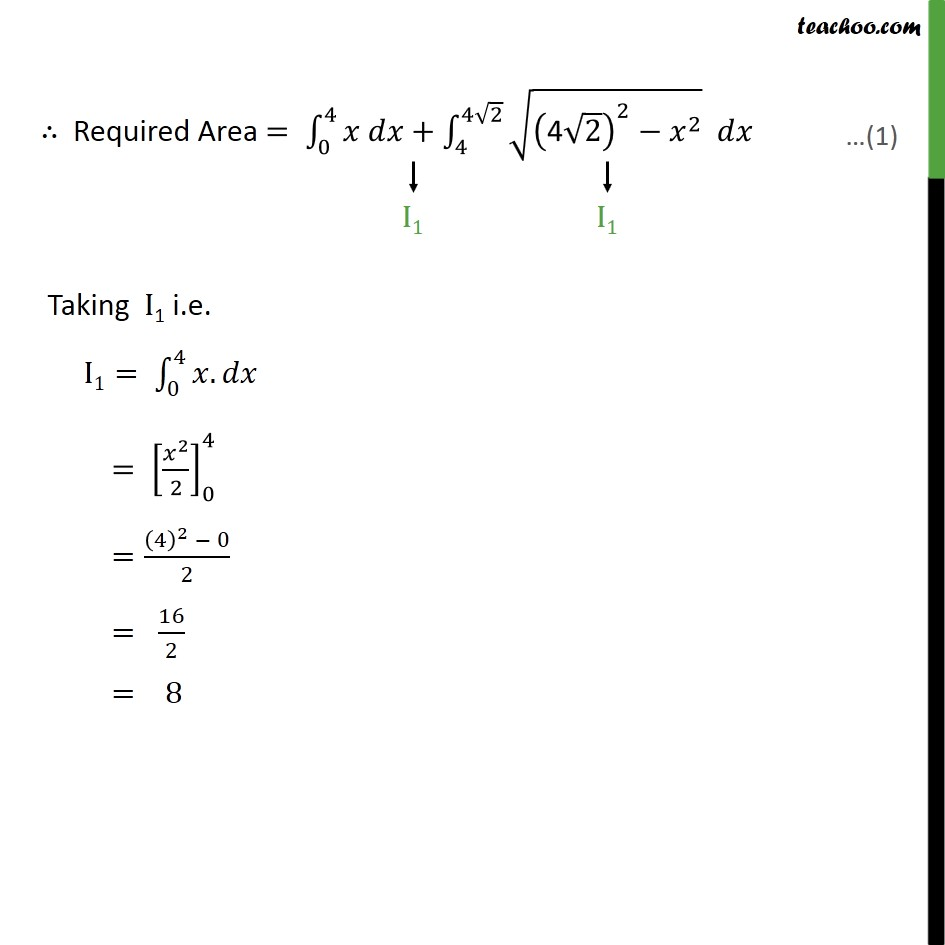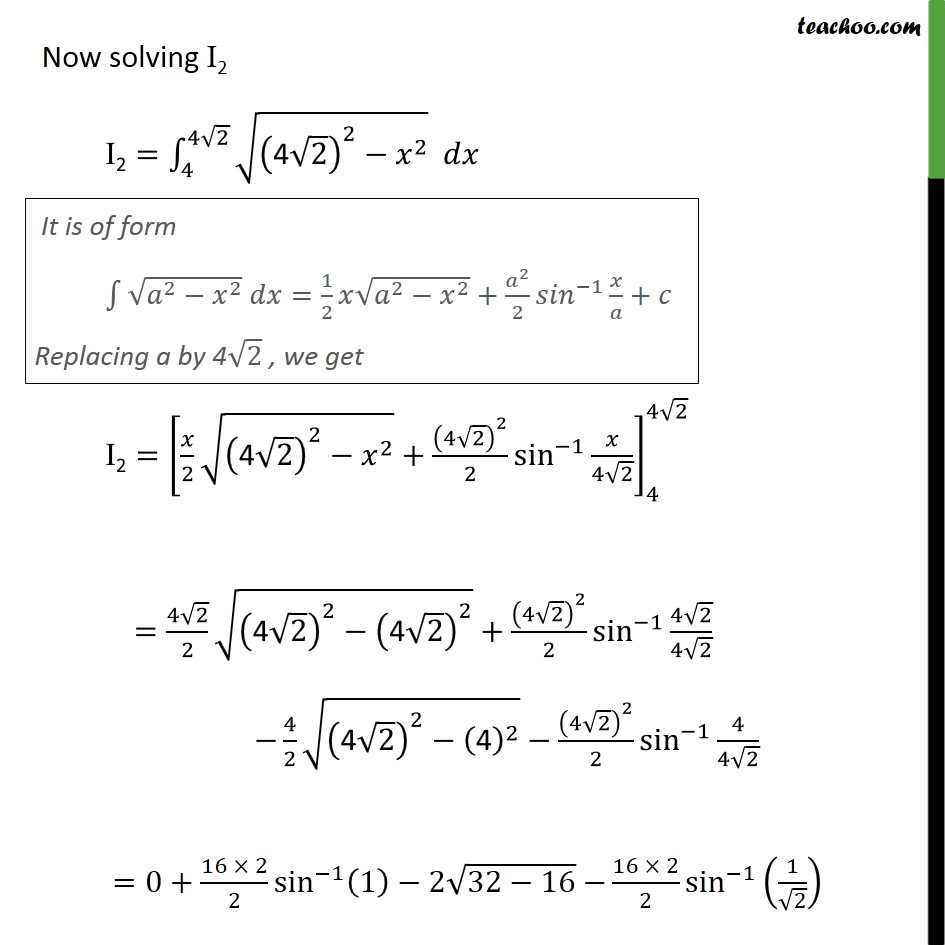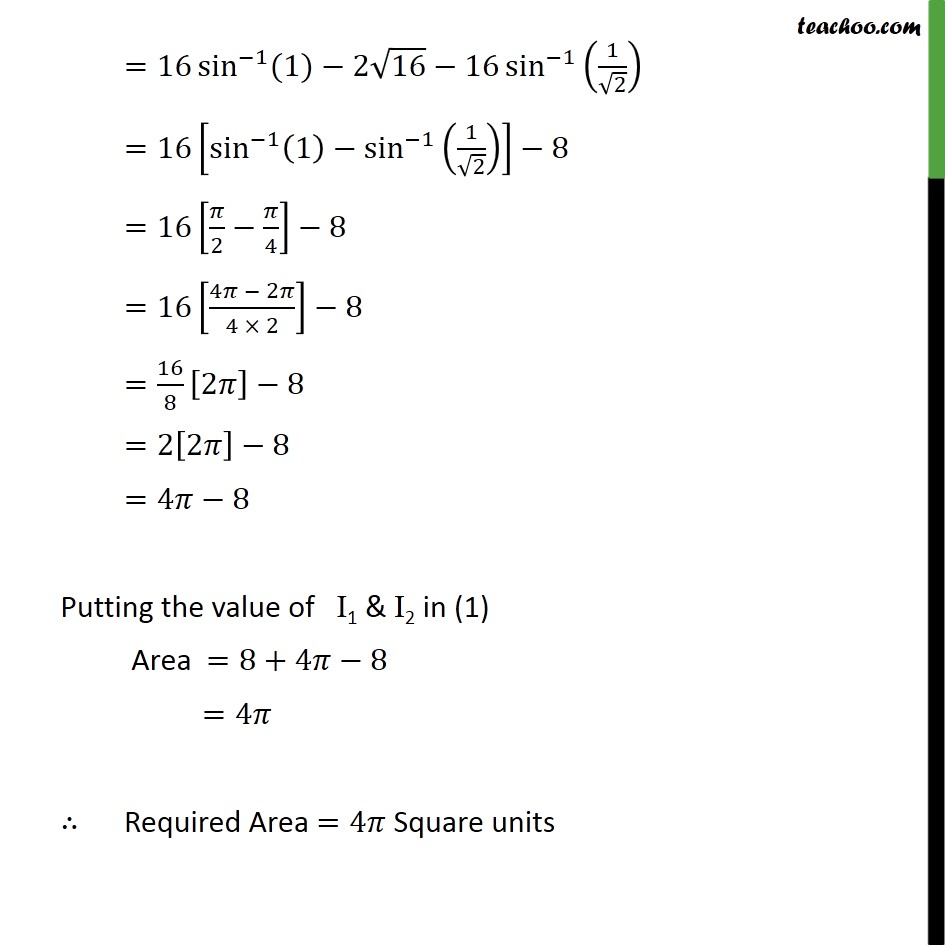Learn in your speed, with individual attention - Teachoo Maths 1-on-1 Class

### Transcript

Question 2 Find the area of the region in the first quadrant enclosed by the -axis, the line = , and the circle 2+ 2=32 Equation of Given Circle is :- 2+ 2=32 2+ 2=16 2 2+ 2= 4 2 2 2+ 2= 4 2 2 Let point where line and circle intersect be point M Required Area = Area of shaded region = Area OMA First we find Point M Point M is intersection of line and circle We know that = Putting this in Equation of Circle, we get 2+ 2=32 2+ 2=32 2 2=32 2=16 = 4 As Point M is in 1st Quadrant M = 4 , 4 Required Area = Area OMP + Area PMA = 0 4 1 + 4 4 2 2 Required Area = 0 4 + 4 4 2 4 2 2 2 Taking I1 i.e. I1= 0 4 . = 2 2 0 4 = 4 2 0 2 = 16 2 = 8 Now solving I2 I2= 4 4 2 4 2 2 2 I2= 2 4 2 2 2 + 4 2 2 2 sin 1 4 2 4 4 2 = 4 2 2 4 2 2 4 2 2 + 4 2 2 2 sin 1 4 2 4 2 4 2 4 2 2 4 2 4 2 2 2 sin 1 4 4 2 =0+ 16 2 2 sin 1 1 2 32 16 16 2 2 sin 1 1 2 =16 sin 1 (1) 2 16 16 sin 1 1 2 =16 sin 1 1 sin 1 1 2 8 =16 2 4 8 =16 4 2 4 2 8 = 16 8 2 8 =2 2 8 =4 8 Putting the value of I1 & I2 in (1) Area =8+4 8 =4 Required Area =4 Square units